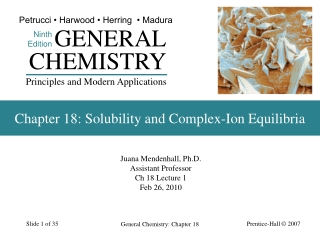DownloadDownload PresentationChapter 18: Solubility and Complex-Ion Equilibria

# Chapter 18: Solubility and Complex-Ion Equilibria

Télécharger la présentation## Chapter 18: Solubility and Complex-Ion Equilibria

- - - - - - - - - - - - - - - - - - - - - - - - - - - E N D - - - - - - - - - - - - - - - - - - - - - - - - - - -
##### Presentation Transcript

1. Petrucci • Harwood • Herring • Madura GENERAL Ninth Edition CHEMISTRY Principles and Modern Applications Chapter 18: Solubility and Complex-Ion Equilibria Juana Mendenhall, Ph.D. Assistant Professor Ch 18 Lecture 1 Feb 26, 2010 General Chemistry: Chapter 18

2. Objectives The Solubility Product Constant, Ksp Write the solubility product expression Ksp for a slightly soluble ionic compound The Relationship Between Solubility and Ksp Calculate the effect of common ions on the aqueous solubilities of sparingly soluble salts The Common-Ion Effect in Solubility Equilibria Determine if a salt will precipitate from solution based on the concentration of its ions General Chemistry: Chapter 18

3. The Solubility Product Constant, Ksp • The equilibrium constant for the equilibrium established between a solid solute and its ions in a saturated solution. CaSO4(s) Ca2+(aq) + SO42-(aq) Ksp = [Ca2+][SO42-] = 9.110-6 at 25°C General Chemistry: Chapter 18

4. Types of Solubility Equilibria General Chemistry: Chapter 18

5. The Relationship Between Solubility and Ksp • Molar solubility. • The molarity in a saturated aqueous solution. • Related to Ksp g BaSO4/100 mL → mol BaSO4/L → [Ba2+] and [SO42-] → Ksp = 1.110-10 General Chemistry: Chapter 18

6. General Chemistry: Chapter 18

7. The Common-Ion Effect in Solubility Equilibria The ion product (Qsp) is related to the solubility product (Ksp) by Qsp < Ksp, more solute can dissolve Qsp > Ksp, precipitate forms If Qsp = Ksp, the solution is saturated General Chemistry: Chapter 18

8. The Common-Ion Effect and Le Châtelier’s Principle General Chemistry: Chapter 18

9. 18-4 Limitations of the Ksp Concept • Ksp is usually limited to slightly soluble solutes. • For more soluble solutes we must use ion activities • Activities (effective concentrations) become smaller than the measured concentrations. • The Salt Effect (or diverse ion effect). • Ionic interactions are important even when an ion is not apparently participating in the equilibrium. • Uncommon ions tend to increase solubility. General Chemistry: Chapter 18

10. Effects on the Solubility of Ag2CrO4 General Chemistry: Chapter 18

11. Ion Pairs General Chemistry: Chapter 18

12. Incomplete Dissociation • Assumption that all ions in solution are completely dissociated is not valid. • Ion Pair formation occurs. • Some solute “molecules” are present in solution. • Increasingly likely as charges on ions increase. Ksp (CaSO4) = 2.310-4 by considering solubility in g/100 mL Table 19: Ksp = 9.110-6 Activities take into account ion pair formation and must be used. General Chemistry: Chapter 18

13. Simultaneous Equilibria • Other equilibria are usually present in a solution. • Kw for example. • These must be taken into account if they affect the equilibrium in question. General Chemistry: Chapter 18

14. 18-5 Criteria for Precipitation and Its Completeness AgI(s) Ag+(aq) + I-(aq) Ksp = [Ag+][Cl-] = 8.510-17 Mix AgNO3(aq) and KI(aq) to obtain a solution that is 0.010 M in Ag+ and 0.015 M in I-. Saturated, supersaturated or unsaturated? Q = [Ag+][Cl-] = (0.010)(0.015) = 1.10-4 > Ksp General Chemistry: Chapter 18

15. The Ion Product Q is generally called the ion product. Q > Ksp Precipitation should occur. Q = Ksp The solution is just saturated. Q < Ksp Precipitation cannot occur. General Chemistry: Chapter 18

16. EXAMPLE 18-5 0.05 mL 1 L 0.20 mol KI 1 mol I- nI- = 3 drops 1 drop 1 L 1 mol KI 1000 mL Applying the Criteria for Precipitation of a Slightly Soluble Solute. Three drops of 0.20 M KI are added to 100.0 mL of 0.010 M Pb(NO3)2. Will a precipitate of lead iodide form? (1 drop  0.05 mL) PbI2(s) → Pb2+(aq) + 2 I-(aq) Ksp= 7.110-9 Determine the amount of I- in the solution: = 310-5 mol I- General Chemistry: Chapter 18

17. EXAMPLE 18-5 310-5 mol I- [I-] = = 310-4 M I- 0.1000 L Determine the concentration of I- in the solution: Apply the Precipitation Criteria: Q = [Pb2+][I-]2 = (0.010)(310-4)2 = 910-10 < Ksp= 7.110-9 General Chemistry: Chapter 18